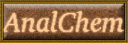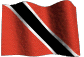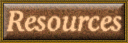### C30F Analytical Chemistry Labscript

A labguide has been produced for each experiment. It gives info on preparations, chemicals, apparatus, and sample requirements. It also includes a helpful analytical notes feature. To get the labguide for this experiment click here Enjoy!

Back to List of Experiments

### Determination of chloride in sea water by ion selective electrode (ISE) using three measurement techniques

1. Introduction:

The operation of the chloride ion selective electrode (ISE) to be used in this experiment is based on the response of a disc of sensing element, which is sealed into one end of an epoxy tube (Bailey 1980).

The tube is filled with an internal filling solution, which contacts the inner surface of the sensing element, as well as an internal electrode which provides a stable electrode potential against which the potential of the sensor element is measured. An external reference electrode is also connected in series with the chloride ISE, and may be incorporated into the ISE with a salt bridge connecting the ISE and reference electrodes. This arrangement, where the ISE and external reference electrodes are housed in a single electrode body is referred to as a combination electrode. (Orion Chloride Combination Electrode Instruction Manual 1990).

When the electrode is placed in a solution containing chloride ions, an electrode potential develops across the sensing element, the magnitude of which depends on the chloride ion activity in the test solution. This potential is measured with a digital mV meter or an ISE meter, and follows the Nernst equation as follows:

E = Eo ± S/n log A where;

E = measured electrode potential (response variable)
Eo= reference electrode potential (constant)
A = chloride ion activity in test solution (independent variable)
S = slope of the electrode (usually 56-60 mV/decade concentration change)
n = charge on ion

For chloride ion, n = -1, so that slope is negative and the equation for the chloride ISE reduces to: E = Eo - S log10 A

where S = slope of the calibration curve (theoretically 59.5mV/decade concentration for a univalent ion)

(A) Standard calibration curve and direct readout by ISE meter:

A plot of E vs log10 A will produce a calibration curve, which will be linear over a range of analyte concentrations, the range depending on the response of the electrode under measurement conditions. Non-linear responses and longer equilibration times are usually observed at low chloride concentrations.

The chloride ion activity (A) in solution is dependent on the ionic activity of the solution and may be related to the total "free" (aquated) chloride ion concentration (Cf) by its ionic activity coefficient γ , according to the following equation: A = γCf

Ionic activity coefficients (γ) are variable and depend heavily on total ionic strength of the solution, which may be calculated as: Ionic strength = 0.5 ΣCiZi˛

where Ci = concentration of ion i and ZI = charge on ion i

If the ionic strength of the test solution can be maintained at a high and almost constant level for both standard and sample solutions, the activity coefficient of the chloride ion in solutions will be similarly constant. Under these conditions, the activity of the chloride ion is directly proportional to the chloride ion concentration in solution.

For this purpose, a constant volume of Ionic Strength Adjuster (ISA), which is a neutral, concentrated, non-interfering salt solution (e.g. 5M sodium nitrate used in this experiment) is added to similar volumes of both samples and standards.

A plot of E vs log10 C of standard chloride solutions thus provides a calibration curve, from which chloride concentrations of samples may be interpolated, based on their respective potentiometric responses. These standards may also be used to calibrate the ISE meter, from which then provides the sample concentrations as direct readout values.

(B) Standard addition for determination of chloride in samples:

The potentiometric response (Ei) of the ISE in a sample solution may be obtained for interpolation from a standard calibration curve as previously described.

If a measured volume (Vs) of a chloride standard solution of concentration (Cs) is added to a known volume (Vo) of the sample solution, then a new response (Ef) is obtained, due to the standard added. The change of chloride concentration (CΔ) in the sample solution is proportional to the change (ΔE = Ef - Ei) in potentiometric response of the electrode (Moody and Thomas 1971).

Where CΔ = (Vs x Cs) / Vo

The original sample concentration (C0) may thus be calculated from the following equation:

Co = CΔ [1/antilog(E/S) - 1]

2. Experimental Procedure:

(A) Preparation of chloride standard solutions in distilled water (no ISA included):

A stock standard 1M chloride solution in distilled water will be provided, as well as a 5M ISA solution. Into 100mL volumetric flasks, pipette 10mL of 1M chloride solution, make to volume with distilled water and mix well. This provides your 10-1M chloride standard solution in distilled water.

Into a second 100mL volumetric flask, pipette 10 mL of the 10-1M chloride standard solution, make to the mark with distilled water and mix well. This provides your 10-2M chloride standard solution in distilled water.

Continue with this serial dilution of the chloride standards to prepare 10-3M, 10-4M, and 10-5M standard solutions respectively in distilled water.

(B) Preparation of chloride calibration standards (ISA included):

Into 100mL volumetric flasks, pipette 10mL of 1M chloride solution, 2 mL of ISA solution, make to volume with distilled water and mix well. This provides your 10-1M chloride standard calibration solution with ISA.

Into a second 100mL volumetric flask, pipette 10 mL of the 10-1M chloride standard solution made up in distilled water only. Add 2 mL of ISA solution, make up to the mark with distilled water and mix well. This provides your 10-2M chloride standard with ISA.

Similarly prepare your 10-3M, 10-4M, and 10-5M chloride standard solutions with ISA.

(C) Determination of chloride in sample provided:

(i) Sample preparation:

A sample of seawater will be provided. Pipette out triplicate 1mL volumes into 100mL volumetric flasks, add 2mL of ISA to each, make to volume with distilled water and mix thoroughly. Prepare a sample blank by adding only the ISA solution and making up to volume with distilled water.

(ii) Procedure for ISE measurements:

When not in use, the sensing element of the chloride ISE sensor should be kept immersed in distilled water. Before placing the electrode in a test solution, rinse the sensing element with distilled water and gently wipe free of water with a strip of clean, absorbent tissue. Be careful not to scratch the sensor membrane surface.

Measurements will be made in 100mL beakers, in which solutions will be stirred using a magnetic stirrer. Pour approximately 50mL of each solution into a beaker, insert a small magnetic stirring bar into the solution, and place the beaker in the center of the magnetic stirring plate. Adjust the speed of rotation of the stirring magnet until the solution is steadily stirred, without creating a vortex.

(iii) Calibration of the ISE meter with chloride standard solutions:

Set up the ISE meter, following the instructions provided with the instrument. Set into direct readout mode, using four standards for calibration.

Carefully lower the electrode into the 10-4M chloride solution, start a stop watch and observe the potentiometric (mV) response, until a stable reading is obtained. Note this mV value and the time taken to achieve it. Enter this reading corresponding to the calibration solution concentration.

Remove the electrode from the calibration solution, rinse with distilled water and lightly wipe with a clean tissue.

Replace the 10-4 standard calibration solution with the 10-3 chloride standard calibration solution and repeat the process, followed by the other 2 standards in increasing order of concentration. Record and tabulate the respective responses and equilibration times of the four standard solutions, for plotting your calibration curve.

The meter is now calibrated for direct readout of chloride concentrations, as well as to provide the corresponding mV values for each solution.

(iv) Analysis of sample solutions:

Pipette 50mL of each test sample into 100mL beakers and measure their chloride concentrations with the calibrated ISE meter. Also note the mV reading of each solution, for interpolation on the calibration curve.

Use the 10-5M chloride standard solution as a sample, to obtain its mV value, for plotting the calibration curve.

Also, immediately after obtaining the initial equilibrated response (Ei) of each test solution, add a calculated volume (<0.5mL) of an appropriate standard, approximately 100x more concentrated than the sample solution concentration (estimated from mV readout and calibration curve - see Demonstrator). Allow to mix into the sample solution and record the new equilibrated mV value (Ef) and time after mixing.

If the response for the sample blank continues to drift for >15 minutes, and gives a direct concentration readout <10-5M chloride, discontinue the measurement and record the reading as << 10-5M.

(D) Calculations:

(i) Standard calibration curve:

Plot log concentration of the 5 chloride standard solutions vs their corresponding mV values.

Use your calibration curve to determine the chloride concentrations of sample solutions.

Using the dilution factors and atomic mass of chloride, calculate the chloride concentration of the seawater sample in parts per thousand (ppt).

Calculate the slope value of the calibration curve, over the linear range of response.

Using the standard equations, calculate the chloride sample concentrations. Convert to ppt concentrations as above.

4. Exercises:

(i) The chloride concentration of the sample, determined by titration, will be provided. Do the results of the three measurement techniques differ significantly (p<.05) from this value?

(2) Explain the reasons for the differences in chloride results among these three measurement techniques.

(3) Suggest three other applications of the chloride ISE.

5. Bibliography:

1. Bailey PL (1980). Analysis with Ion-Selective Electrodes, Second Edition, Heyden (London UK) 80-124

2. Moody GJ and Thomas JDR (1971). Selective Ion Sensitive Electrodes, Merrow (Bath, UK) 50-55

3. Orion Combination Chloride Electrode Instruction Manual. Model 94-17B (1990) Orion Research Inc.(Mass., USA) 1-30

Anal-Chem Resources
delloyd.50megs.com
Chem. Dept. UWI. St. Augustine Campus